Updating search results...

# 13 Results

View
Selected filters:
• MCCRS.Math.Content.7.EE.B.4b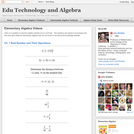Conditional Remix & Share Permitted
CC BY-NC
Rating
0.0 stars

Visually searchable database of Algebra 1 videos. Click on a problem and watch the solution on YouTube. Copy and paste this material into your CMS. Videos accompany the open Elementary Algebra textbook published by Flat World Knowledge.

Subject:
Algebra
Mathematics
Material Type:
Lecture
Lecture Notes
Provider:
Individual Authors
Provider Set:
Individual Authors
Author:
John Redden
04/29/2012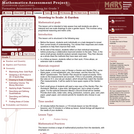Only Sharing Permitted
CC BY-NC-ND
Rating
0.0 stars

This lesson unit is intended to help assess how well students are able to interpret and use scale drawings to plan a garden layout. This involves using proportional reasoning and metric units.

Subject:
Algebra
Geometry
Mathematics
Ratios and Proportions
Material Type:
Assessment
Lesson Plan
Provider:
Shell Center for Mathematical Education
Provider Set:
Mathematics Assessment Project (MAP)
04/26/2013Unrestricted Use
CC BY
Rating
0.0 stars

This task is the second in a series of three tasks that use inequalities in the same context at increasing complexity in 6th grade, 7th grade and in HS algebra. Students write and solve inequalities, and represent the solutions graphically.

Subject:
Algebra
Mathematics
Material Type:
Activity/Lab
Provider:
Illustrative Mathematics
Provider Set:
Illustrative Mathematics
Author:
Illustrative Mathematics
05/01/2012Unrestricted Use
CC BY
Rating
0.0 stars

This problem asks the students to represent a sequence of operations using an expression and then to write and solve simple equations. The problem is posed as a game and allows the students to visualize mathematical operations. It would make sense to actually play a similar game in pairs first and then ask the students to record the operations to figure out each other's numbers.

Subject:
Algebra
Mathematics
Material Type:
Activity/Lab
Provider:
Illustrative Mathematics
Provider Set:
Illustrative Mathematics
Author:
Illustrative Mathematics
08/07/2012Conditional Remix & Share Permitted
CC BY-NC
Rating
0.0 stars

Four full-year digital course, built from the ground up and fully-aligned to the Common Core State Standards, for 7th grade Mathematics. Created using research-based approaches to teaching and learning, the Open Access Common Core Course for Mathematics&nbsp;is designed with student-centered learning in mind, including activities for students to develop valuable 21st century skills and academic mindset.

Subject:
Mathematics
Material Type:
Full Course
Provider:
Pearson
10/06/2016Conditional Remix & Share Permitted
CC BY-NC
Rating
0.0 stars

Algebraic Reasoning

Type of Unit: Concept

Prior Knowledge

Students should be able to:

Add, subtract, multiply, and divide rational numbers.
Evaluate expressions for a value of a variable.
Use the distributive property to generate equivalent expressions including combining like terms.
Understand solving an equation or inequality as a process of answering a question: which values from a specified set, if any, make the equation or inequality true?
Write and solve equations of the form x+p=q and px=q for cases in which p, q, and x are non-negative rational numbers.
Understand and graph solutions to inequalities x&lt;c or x&gt;c.
Use equations, tables, and graphs to represent the relationship between two variables.
Relate fractions, decimals, and percents.
Solve percent problems included those involving percent of increase or percent of decrease.

Lesson Flow

This unit covers all of the Common Core State Standards for Expressions and Equations in Grade 7. Students extend what they learned in Grade 6 about evaluating expressions and using properties to write equivalent expressions. They write, evaluate, and simplify expressions that now contain both positive and negative rational numbers. They write algebraic expressions for problem situations and discuss how different equivalent expressions can be used to represent different ways of solving the same problem. They make connections between various forms of rational numbers. Students apply what they learned in Grade 6 about solving equations such as x+2=6 or 3x=12 to solving equations such as 3x+6=12 and 3(x−2)=12. Students solve these equations using formal algebraic methods. The numbers in these equations can now be rational numbers. They use estimation and mental math to estimate solutions. They learn how solving linear inequalities differs from solving linear equations and then they solve and graph linear inequalities such as −3x+4&lt;12. Students use inequalities to solve real-world problems, solving the problem first by arithmetic and then by writing and solving an inequality. They see that the solution of the algebraic inequality may differ from the solution to the problem.

Subject:
Algebra
Mathematics
Material Type:
Unit of Study
Provider:
PearsonConditional Remix & Share Permitted
CC BY-NC
Rating
0.0 stars

Students write and solve inequalities in order to solve two problems. One of the problems is a real-world problem that involves selling a house and paying the real estate agent a commission. The second problem involves the relationship of the lengths of the sides of a triangle.Key ConceptsIn this lesson, students again use algebraic inequalities to solve word problems, including real-world situations. Students represent a quantity with a variable, write an inequality to solve the problem, use the properties of inequality to solve the inequality, express the solution in words, and make sure that the solution makes sense.Students explore the relationships of the lengths of the sides of a triangle. They apply the knowledge that the sum of the lengths of any two sides of a triangle must be greater than the length of the third side to solve for the lengths of sides of a triangle using inequalities. They solve the inequality for the length of the third side.Goals and Learning ObjectivesUse an algebraic inequality to solve problems, including real-world problems.Use the properties of inequalities to solve an inequality.

Subject:
Algebra
Geometry
Material Type:
Lesson Plan
09/21/2015Conditional Remix & Share Permitted
CC BY-NC
Rating
0.0 stars

Students work in pairs to critique and improve their work on the Self Check. Students complete a task similar to the Self Check with a partner.Key ConceptsTo critique and improve the task from the Self Check and to complete a similar task with a partner, students use what they know about solving inequalities, graphing their solutions, and relating the inequalities to a real-world situation.Goals and Learning ObjectivesSolve algebraic inequalities.Graph the solutions of inequalities using number lines.Write word problems that match algebraic inequalities.Interpret the solution of an inequality in terms of a word problem.

Subject:
Algebra
Material Type:
Lesson Plan
09/21/2015Conditional Remix & Share Permitted
CC BY-NC
Rating
0.0 stars

Students discover how the addition and multiplication properties of inequality differ from the addition and multiplication properties of equality.Students use the addition and multiplication properties of inequality to solve inequalities. They graph their solutions on the number line.Key ConceptsIn this lesson, students extend their knowledge of inequalities from Grade 6. In Grade 6, students learned that solving an inequality meant finding which values made the inequality true. Students used substitution to determine whether a given value made an inequality true. They also used a number line to graph the solutions of inequalities. By graphing these solutions on a number line, they saw that an inequality has an infinite number of solutions.Now, in Grade 7, students work with inequalities that also contain negative numbers and learn to solve and graph solutions for inequalities such as &minus;2x &minus; 4 &lt; 5. This involves first understanding how the properties of inequality differ from the properties of equality. When multiplying (or dividing) both sides of an inequality by the same negative number, the relationship between the two sides of the inequality changes, so it is necessary to reverse the direction of the inequality sign in order for the inequality to remain true. Once students understand this, they can apply the same steps they used to solve equations to solve inequalities, but remembering to reverse the direction of the inequality sign when multiplying or dividing both sides of the inequality by a negative number.Goals and Learning ObjectivesAccess prior knowledge of how to solve an inequality.Observe that when multiplying or dividing both sides of an inequality by the same negative number, the inequality sign must change direction.Solve and graph inequalities of the form px + q &gt; r or px + q &lt; r, where p, q, and r are specific rational numbers.

Subject:
Algebra
Material Type:
Lesson Plan
09/21/2015Conditional Remix & Share Permitted
CC BY-NC
Rating
0.0 stars

Students solve a problem about a salesperson&#39;s compensation. They solve the problem first by arithmetic and then by writing and solving an inequality.Key ConceptsIn Lesson 11, students learned how to solve inequalities using the addition and multiplication properties of inequality. In this lesson, they solve word problems by writing and solving inequalities.To help students make connections and see how problems can be solved in different ways, students first solve the same problem using arithmetic.Goals and Learning ObjectivesWrite and solve an algebraic inequality to solve a word problem.

Subject:
Algebra
Material Type:
Lesson Plan
09/21/2015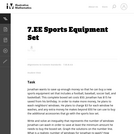Unrestricted Use
CC BY
Rating
0.0 stars

The purpose of this task is to present students with a context that can naturally be represented with an inequality and to explore the relationship between the context and the mathematical representation of that context; thus, this is an intended as an instructional task.

Subject:
Mathematics
Material Type:
Activity/Lab
Provider:
Illustrative Mathematics
Provider Set:
Illustrative Mathematics
Author:
Illustrative Mathematics
08/10/2012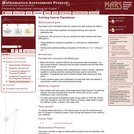Only Sharing Permitted
CC BY-NC-ND
Rating
0.0 stars

This lesson unit is intended to help teachers assess how well students are able to: form and solve linear equations involving factorizing and using the distributive law. In particular, this unit aims to help teachers identify and assist students who have difficulties in: using variables to represent quantities in a real-world or mathematical problem and solving word problems leading to equations of the form px + q = r and p(x + q) = r.

Subject:
Algebra
Mathematics
Material Type:
Assessment
Lesson Plan
Provider:
Shell Center for Mathematical Education
Provider Set:
Mathematics Assessment Project (MAP)
04/26/2013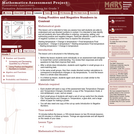Only Sharing Permitted
CC BY-NC-ND
Rating
0.0 stars

This lesson unit is intended to help teachers assess how well students are able to understand and use directed numbers in context. It is intended to help identify and aid students who have difficulties in ordering, comparing, adding, and subtracting positive and negative integers. Particular attention is paid to the use of negative numbers on number lines to explore the structures: starting temperature + change in temperature = final temperature final temperature Đ change in temperature = starting temperature final temperature Đ starting temperature = change in temperature.

Subject:
Mathematics
Numbers and Operations
Material Type:
Assessment
Lesson Plan
Provider:
Shell Center for Mathematical Education
Provider Set:
Mathematics Assessment Project (MAP)